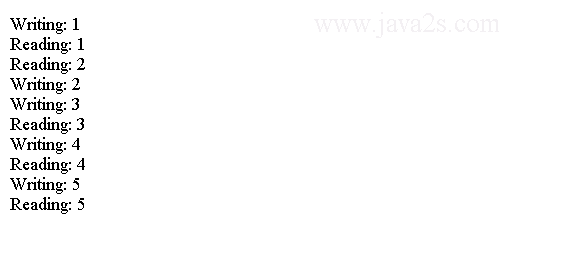# Java IO Tutorial - Java Pipe

A pipe connects an input stream and an output stream.

A piped I/O is based on the producer-consumer pattern, where the producer produces data and the consumer consumes the data.

In a piped I/O, we create two streams representing two ends of the pipe. A PipedOutputStream object represents one end and a PipedInputStream object represents the other end. We connect the two ends using the connect() method on the either object.

We can also connect them by passing one object to the constructor when we create another object.

The following code shows two ways of creating and connecting the two ends of a pipe:

The first method creates a piped input and output streams and connect them. It connects the two streams using `connect` method.

```PipedInputStream pis  = new PipedInputStream();
PipedOutputStream pos  = new PipedOutputStream();
pis.connect(pos); /* Connect  the   two  ends  */
```

The second method creates piped input and output streams and connect them. It connects the two streams by passing the input piped stream to the output stream constructor.

```PipedInputStream pis  = new PipedInputStream();
PipedOutputStream pos  = new PipedOutputStream(pis);
```

We can produce and consume data after we connect the two ends of the pipe.

We produce data by using one of the write() methods of the PipedOutputStream object. Whatever we write to the piped output stream automatically becomes available to the piped input stream object for reading.

We use the read() method of PipedInputStream to read data from the pipe. The piped input stream is blocked if data is not available when it attempts to read from the pipe.

A piped stream has a buffer with a fixed capacity to store data between the time it is written to and read from the pipe.

We can set the pipe capacity when we create it. If a pipe's buffer is full, an attempt to write on the pipe will block.

The following code creates piped input and output streams with the buffer capacity of 2048 bytes.

```PipedOutputStream pos  = new PipedOutputStream();
PipedInputStream pis  = new PipedInputStream(pos, 2048);
```

A pipe is used to transfer data from one thread to another. The synchronization between two threads is taken care of by the blocking read and write.

## Example

The following code demonstrates how to use a piped I/O.

```import java.io.PipedInputStream;
import java.io.PipedOutputStream;
//w w w. j av  a  2 s.c  o m
public class Main {
public static void main(String[] args) throws Exception {
PipedInputStream pis = new PipedInputStream();
PipedOutputStream pos = new PipedOutputStream();
pos.connect(pis);

Runnable producer = () -> produceData(pos);
Runnable consumer = () -> consumeData(pis);
}

public static void produceData(PipedOutputStream pos) {
try {
for (int i = 1; i <= 50; i++) {
pos.write((byte) i);
pos.flush();
System.out.println("Writing: " + i);
}
pos.close();
} catch (Exception e) {
e.printStackTrace();
}
}
public static void consumeData(PipedInputStream pis) {
try {
int num = -1;
while ((num = pis.read()) != -1) {